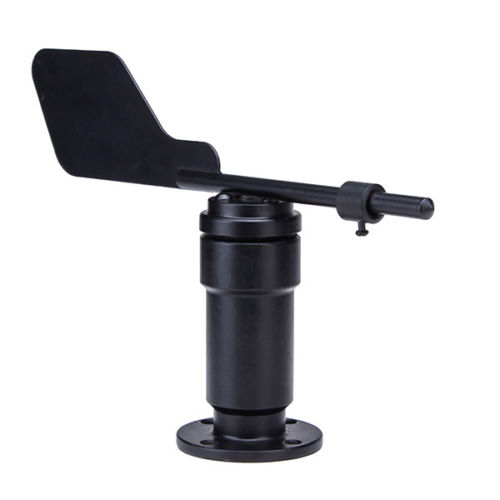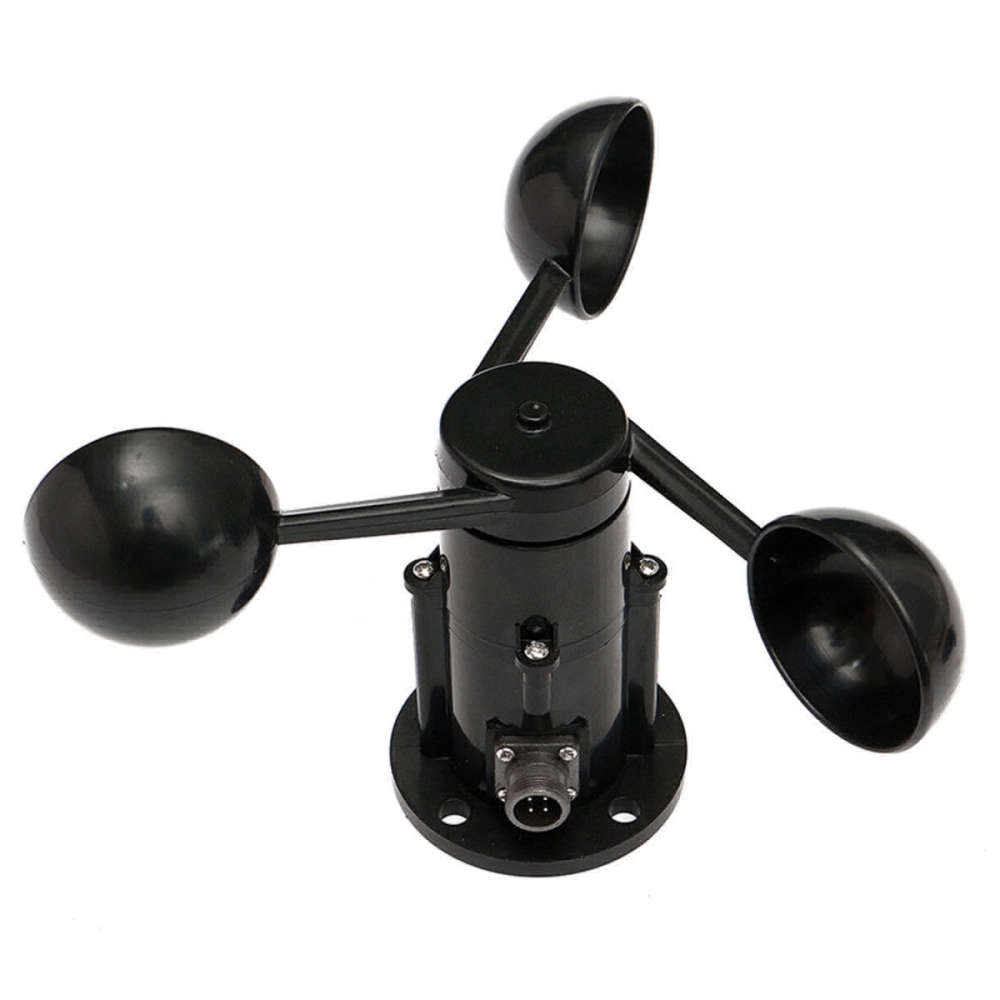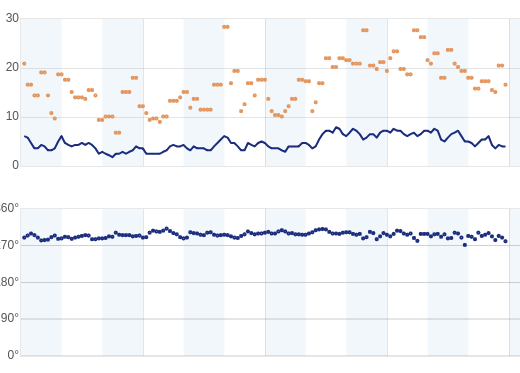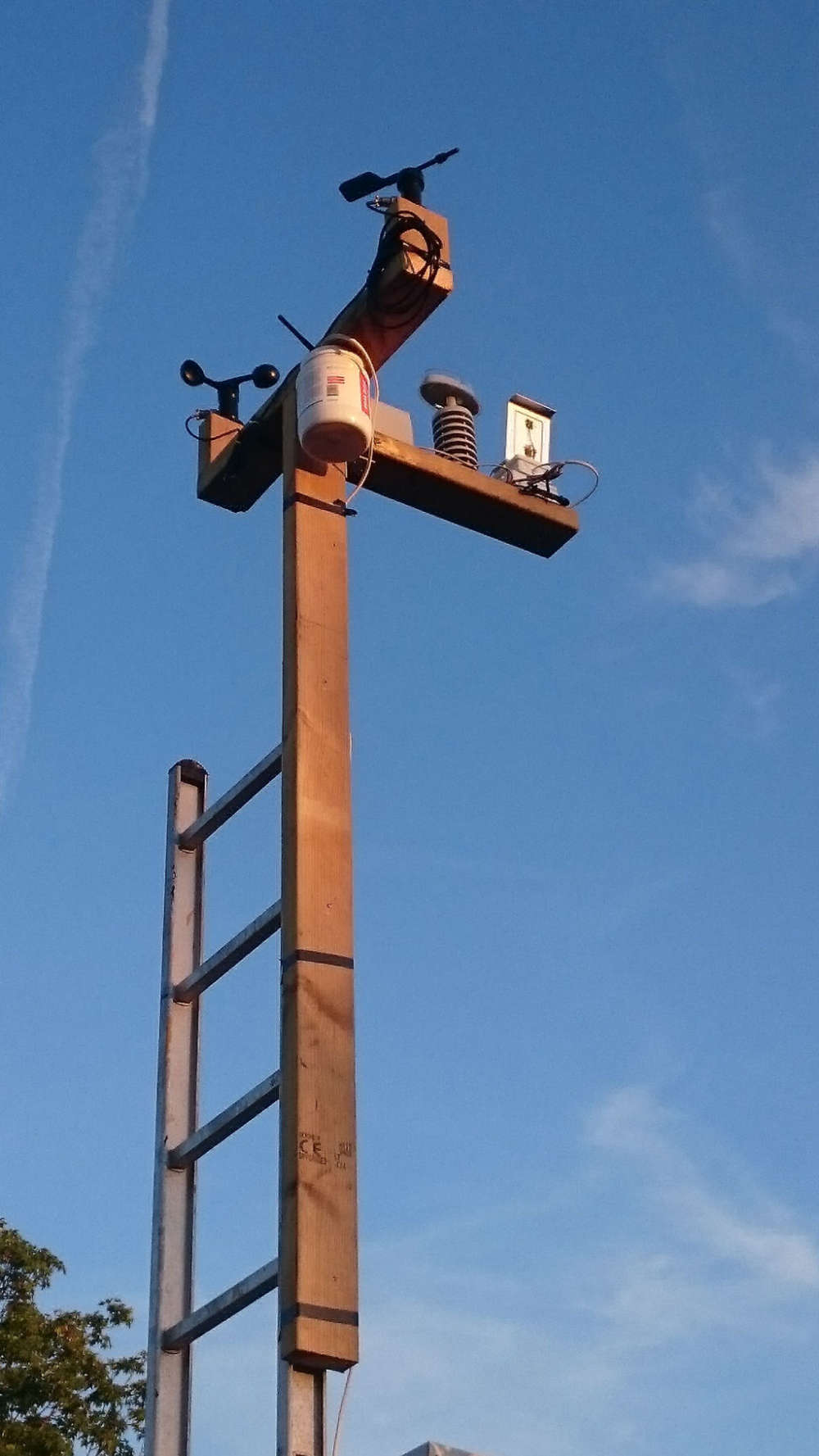# My Wind Station

• I made a wind station using the two following sensors that I bought on e-bay.

• Wind Sensor JL-FS2 Wind Direction Sensor (4-20mA, 0-5V) Signal Output
• Wind Speed Sensor Anemometer Three Cups Aluminium Alloyed pulse signal outputI think they are quite nice. They are fed with 12V DC and are giving a 5V output signal. Therefore I'm using a 5V Arduino Mini Pro for the sketch.

The following sketch is measuring the wind data once a second, storing it into an array and doing calculations to get the average wind speed, direction and gust for each minute, each other minute and each 10 minutes.

Before getting the average the wind is split into it's vector components. Cool isn't it?

I upload the average 10 minute wind data to Weather Underground. It looks very smooth.

Here is the sketch:

``````/**
* WindMonitor
*

About the windspeed sensor, have a look here:
https://www.dfrobot.com/wiki/index.php/Wind_Speed_Sensor_Voltage_Type(0-5V)_SKU:SEN0170#Connection_Diagram

*/
#define MY_NODE_ID 17

#define SKETCH_NAME "Wind Station"
#define SKETCH_VERSION "1.1.1"
#define DWELL_TIME 1000  // this allows for radio to come back to power after a transmission, ideally 0

//#define MY_DEBUG // Enable debug Serial.prints to serial monitor and arrays are smaller

#if defined MY_DEBUG
#define MY_BAUD_RATE 115200 // Sets the serial baud rate for console and serial gateway

#define Sprintln(a) (Serial.println(a))
#define Sprint(a) (Serial.print(a))
const uint8_t num1mReadings = 2; // Use a low value to free memory for making debug possible
const uint8_t num2mReadings = 2; // Use a low value to free memory for making debug possible
const uint8_t num10mReadings = 2; // Use a low value to free memory for making debug possible
const uint8_t FORCE_TRANSMIT_CYCLE = 10; // Force sending value even if hasn't changed after N cycles
const uint16_t WAIT_TIME = 5000;
#else
#define Sprintln(a)
#define Sprint(a)
const uint8_t num1mReadings = 60; //Defines number of reading to calculate average windspeed
const uint8_t FORCE_TRANSMIT_CYCLE = 10; // Force sending value even if hasn't changed after N cycles
const uint16_t WAIT_TIME = 1000;
#endif

// Enable and select radio type attached
//#define MY_PARENT_NODE_ID 0
//#define MY_PARENT_NODE_IS_STATIC
#include <MySensors.h>

#define LED_PIN 8
#define ANEMOMETER_ANALOG_PIN 0
#define WIND_VANE_ANALOG_PIN 1

#define CHILD_ID_WIND 0
#define CHILD_ID_WIND2M 1
#define CHILD_ID_WIND10M 2
#define CHILD_ID_GUST_DIR 3 // Specifically for wind gust direction
#define CHILD_ID_GUST2M_DIR 4
#define CHILD_ID_GUST10M_DIR 5

#define FORCE_TRANSMIT_CYCLE 60 // Force sending value even if hasn't changed after N cycles
uint8_t cycleCountWindSpeed = FORCE_TRANSMIT_CYCLE;
uint8_t cycleCountWindGust = FORCE_TRANSMIT_CYCLE;
uint8_t cycleCountWindGustDir = FORCE_TRANSMIT_CYCLE;
uint8_t cycleCountWindDir = FORCE_TRANSMIT_CYCLE;
uint8_t cycleCountWindSpeed2m = FORCE_TRANSMIT_CYCLE;
uint8_t cycleCountWindGust2m = FORCE_TRANSMIT_CYCLE;
uint8_t cycleCountWindGustDir2m = FORCE_TRANSMIT_CYCLE;
uint8_t cycleCountWindDir2m = FORCE_TRANSMIT_CYCLE;
uint8_t cycleCountWindSpeed10m = FORCE_TRANSMIT_CYCLE;
uint8_t cycleCountWindGust10m = FORCE_TRANSMIT_CYCLE;
uint8_t cycleCountWindGustDir10m = FORCE_TRANSMIT_CYCLE;
uint8_t cycleCountWindDir10m = FORCE_TRANSMIT_CYCLE;

float last_wspeed;
float last_wgust;
uint16_t last_wdirection;
uint16_t last_wgustdirection;
float last_wspeed2m;
float last_wgust2m;
uint16_t last_wdirection2m;
uint16_t last_wgustdirection2m;
float last_wspeed10m;
float last_wgust10m;
uint16_t last_wdirection10m;
uint16_t last_wgustdirection10m;

boolean got2mins = false;
boolean got10mins = false;

float windSpeed = 0.0; // Wind speed in meters per second (m/s)
float windDir = 0; // Wind dir in degrees 0-360
uint8_t minute2Index = 0; // the index of the current reading
uint8_t minute10Index = 0; // the index of the current reading
uint16_t sensorValue = 0; //Variable stores the value direct from the analog pin
const uint8_t wsMin = 22; // Mininum reading
const uint16_t wsMax = 1023; // Maximum reading
const uint8_t wdMin = 3; // Mininum reading
const uint16_t wdMax = 941; // Maximum reading
const uint8_t windSpeedMax = 30; // Wind speed in meters/sec corresponding to maximum voltage

MyMessage msgWSpeed(CHILD_ID_WIND, V_WIND);
MyMessage msgWGust(CHILD_ID_WIND, V_GUST);
MyMessage msgWDirection(CHILD_ID_WIND, V_DIRECTION);
MyMessage msgWSpeed2m(CHILD_ID_WIND2M, V_WIND);
MyMessage msgWGust2m(CHILD_ID_WIND2M, V_GUST);
MyMessage msgWDirection2m(CHILD_ID_WIND2M, V_DIRECTION);
MyMessage msgWSpeed10m(CHILD_ID_WIND10M, V_WIND);
MyMessage msgWGust10m(CHILD_ID_WIND10M, V_GUST);
MyMessage msgWDirection10m(CHILD_ID_WIND10M, V_DIRECTION);
MyMessage msgWGustDir(CHILD_ID_GUST_DIR, V_DIRECTION);
MyMessage msgWGust2mDir(CHILD_ID_GUST2M_DIR, V_DIRECTION);
MyMessage msgWGust10mDir(CHILD_ID_GUST10M_DIR, V_DIRECTION);

void setup()
{
Sprint(SKETCH_NAME);
Sprint(F(" version "));
Sprint(SKETCH_VERSION);
Sprint(F(" (using MY_NODE_ID: "));
Sprint(MY_NODE_ID);
Sprintln(F(") says hello!"));

// Initialize arrays
}
for (minute2Index = 0; minute2Index < num2mReadings; minute2Index++) {
}
for (minute10Index = 0; minute10Index < num10mReadings; minute10Index++) {
}
}

void presentation()  {
// Send the sketch version information to the gateway and Controller
sendSketchInfo(SKETCH_NAME, SKETCH_VERSION);
wait(DWELL_TIME);
// Register all sensors to gateway (they will be created as child devices)
present(CHILD_ID_WIND, S_WIND);
wait(DWELL_TIME);
present(CHILD_ID_WIND2M, S_WIND);
wait(DWELL_TIME);
present(CHILD_ID_WIND10M, S_WIND);
wait(DWELL_TIME);
present(CHILD_ID_GUST_DIR, S_WIND);
wait(DWELL_TIME);
present(CHILD_ID_GUST2M_DIR, S_WIND);
wait(DWELL_TIME);
present(CHILD_ID_GUST10M_DIR, S_WIND);
wait(DWELL_TIME);
}

struct speedAndDir {
float uv;
float Dv;
};

struct speedAndDir windvec(float speedArr[], float dirArr[], uint8_t size) {
/*
*
*       Function to calculate the wind vector from time series of wind speed and direction.
*
*       Parameters:
*           - u: array of wind speeds [m s-1].
*           - D: array of wind directions [degrees from North].
*
*       Returning speedAndDir struct values:
*           - uv: Vector wind speed [m s-1].
*           - Dv: Vector wind direction [degrees from North].
*
*/
struct speedAndDir o;
float ve = 0.0; // define east component of wind speed
float vn = 0.0; // define north component of wind speed

for (int i = 0; i < size; ++i) {
ve += speedArr[i] * sin(dirArr[i] * PI / 180.0); // calculate sum east speed components
vn += speedArr[i] * cos(dirArr[i] * PI / 180.0); // calculate sum north speed components
}
ve = - ve / size; // determine average east speed component
vn = - vn / size; // determine average north speed component
o.uv = sqrt(ve * ve + vn * vn); // calculate wind speed vector magnitude
// Calculate wind speed vector direction
float vdir = atan2(ve, vn);
vdir = vdir * 180.0 / PI; // Convert radians to degrees
if (vdir < 180) {
o.Dv = vdir + 180.0;
}
else {
if (vdir > 180.0) {
o.Dv = vdir - 180;
}
else {
o.Dv = vdir;
}
}
o.uv = ((int) (o.uv * 10.0 + 0.5) / 10.0); // Round to one decimal
o.Dv = (o.uv == 0.0) ? 0 : ((int) (o.Dv + 0.5)); // Round to integer
return o;
}

void loop()
{
}

/*
*/
sensorValue = analogRead(ANEMOMETER_ANALOG_PIN); //Get a value between 0 and 1023 from the analog pin connected to the anemometer
sensorValue = (sensorValue < wsMin) ? wsMin : sensorValue;
windSpeed = (float)(sensorValue - wsMin)*windSpeedMax/(wsMax - wsMin);

Sprint(F(", Wind speed sensor value: "));
Sprint(sensorValue);
Sprint(F(", wind speed: "));
Sprint(windSpeed);
Sprint(F(" m/s."));

/*
*/
const float dirTable[] PROGMEM = {0.0, 22.5, 45.0, 67.5, 90.0, 112.5, 135.0, 157.5, 180.0, 202.5, 225.0, 247.5, 270.0, 292.5, 315.0, 337.5};
sensorValue = (windSpeed > 0) ? analogRead(WIND_VANE_ANALOG_PIN) : wdMin; //Get a value between 0 and 1023 from the analog pin connected to the wind vane
sensorValue = (sensorValue < wdMin) ? wdMin : sensorValue;

windDir = (sensorValue - wdMin)*337.5/(wdMax - wdMin); // This will be close but not exact.
// Find the closest of the 16 predefined wind directions
for (int i = 0; i < 16; ++i)
{
if (windDir < (dirTable[i] + 11.25)) {
windDir = dirTable[i];
break;
}
}

Sprint(F("\tWind direction sensor value: "));
Sprint(sensorValue);
Sprint(F(", wind direction: "));
Sprint(windDir);
Sprintln(F("°"));

minute2Index++;
minute2Index = (minute2Index >= num2mReadings) ? 0 : minute2Index;
minute10Index++;
minute10Index = (minute10Index >= num10mReadings) ? 0 : minute10Index;
got2mins = (minute2Index == num2mReadings-1) ? true : got2mins; // One time switch
got10mins = (minute10Index == num10mReadings-1) ? true : got10mins;

cycleCountWindSpeed++;
cycleCountWindGust++;
cycleCountWindGustDir++;
cycleCountWindDir++;
cycleCountWindSpeed2m++;
cycleCountWindGust2m++;
cycleCountWindGustDir2m++;
cycleCountWindDir2m++;
cycleCountWindSpeed10m++;
cycleCountWindGust10m++;
cycleCountWindGustDir10m++;
cycleCountWindDir10m++;

struct speedAndDir o;
Sprint(F("\nWind vector calculation results: Speed: "));
Sprint(o.uv);
Sprint(F(" m/s"));
Sprint(F(" \tDirection: "));
Sprint(o.Dv);
Sprintln(F("°\n"));

float gust = 0;
uint16_t gustDir = 0;
for (int i = 0; i < num1mReadings; ++i)
{
}
gust = ((int) (gust * 10.0 + 0.5) / 10.0);

if ((o.uv != last_wspeed) or (cycleCountWindSpeed >= FORCE_TRANSMIT_CYCLE)) {
cycleCountWindSpeed = 0;
last_wspeed = o.uv;
Sprint(F("Sending wind speed: "));
Sprint(o.uv);
Sprintln(F(" m/s"));
send(msgWSpeed.set(o.uv, 1));
wait(DWELL_TIME);
}
if ((gust != last_wgust) or (cycleCountWindGust >= FORCE_TRANSMIT_CYCLE)) {
cycleCountWindGust = 0;
last_wgust = gust;
Sprint(F("Sending wind gust speed: "));
Sprint(gust);
Sprintln(F(" m/s"));
send(msgWGust.set(gust, 1));
wait(DWELL_TIME);
}
if ((o.Dv != last_wdirection) or (cycleCountWindDir >= FORCE_TRANSMIT_CYCLE)) {
cycleCountWindDir = 0;
last_wdirection = o.Dv;
Sprint(F("Sending wind direction: "));
Sprint(o.Dv);
Sprintln(F("°"));
send(msgWDirection.set(o.Dv, 0));
wait(DWELL_TIME);
}
if ((gustDir != last_wgustdirection) or (cycleCountWindGustDir >= FORCE_TRANSMIT_CYCLE)) {
cycleCountWindGustDir = 0;
last_wgustdirection = gustDir;
Sprint(F("Sending wind gust direction: "));
Sprint(gustDir);
Sprintln(F("°"));
send(msgWGustDir.set(gustDir, 0));
wait(DWELL_TIME);
}
// Do the 2 minute average but only if we have been running for 2 minutes or more.
if (got2mins) {
Sprint(F("\n==========2 minute wind vector calculation results: Speed: "));
Sprint(o.uv);
Sprint(F(" m/s"));
Sprint(F(" \tDirection: "));
Sprint(o.Dv);
Sprintln(F("°\n"));

gust = gustDir = 0;
for (int i = 0; i < num2mReadings; ++i)
{
}
gust = ((int) (gust * 10.0 + 0.5) / 10.0);

if ((o.uv != last_wspeed2m) or (cycleCountWindSpeed2m >= FORCE_TRANSMIT_CYCLE)) {
cycleCountWindSpeed2m = 0;
last_wspeed2m = o.uv;
Sprint(F("Sending 2 min wind speed: "));
Sprint(o.uv);
Sprintln(F(" m/s"));
send(msgWSpeed2m.set(o.uv, 1));
wait(DWELL_TIME);
}
if ((gust != last_wgust2m) or (cycleCountWindGust2m >= FORCE_TRANSMIT_CYCLE)) {
cycleCountWindGust2m = 0;
last_wgust2m = gust;
Sprint(F("Sending 2 min wind gust speed: "));
Sprint(gust);
Sprintln(F(" m/s"));
send(msgWGust2m.set(gust, 1));
wait(DWELL_TIME);
}
if ((o.Dv != last_wdirection2m) or (cycleCountWindDir2m >= FORCE_TRANSMIT_CYCLE)) {
cycleCountWindDir2m = 0;
last_wdirection2m = o.Dv;
Sprint(F("Sending 2 min wind direction: "));
Sprint(o.Dv);
Sprintln(F("°"));
send(msgWDirection2m.set(o.Dv, 0));
wait(DWELL_TIME);
}
if ((gustDir != last_wgustdirection2m) or (cycleCountWindGustDir2m >= FORCE_TRANSMIT_CYCLE)) {
cycleCountWindGustDir2m = 0;
last_wgustdirection2m = gustDir;
Sprint(F("Sending 2 min wind gust direction: "));
Sprint(gustDir);
Sprintln(F("°"));
send(msgWGust2mDir.set(gustDir, 0));
wait(DWELL_TIME);
}
}

// Do the 10 minute average but only if we have been running for 10 minutes or more.
if (got10mins) {
Sprint(F("\n#############10 minute wind vector calculation results: Speed: "));
Sprint(o.uv);
Sprint(F(" m/s"));
Sprint(F(" \tDirection: "));
Sprint(o.Dv);
Sprintln(F("°\n"));

gust = gustDir = 0;
for (int i = 0; i < num10mReadings; ++i)
{
}
gust = ((int) (gust * 10.0 + 0.5) / 10.0);

if ((o.uv != last_wspeed10m) or (cycleCountWindSpeed10m >= FORCE_TRANSMIT_CYCLE)) {
cycleCountWindSpeed10m = 0;
last_wspeed10m = o.uv;
Sprint(F("Sending 10 min wind speed: "));
Sprint(o.uv);
Sprintln(F(" m/s"));
send(msgWSpeed10m.set(o.uv, 1));
wait(DWELL_TIME);
}
if ((gust != last_wgust10m) or (cycleCountWindGust10m >= FORCE_TRANSMIT_CYCLE)) {
cycleCountWindGust10m = 0;
last_wgust10m = gust;
Sprint(F("Sending 10 min wind gust speed: "));
Sprint(gust);
Sprintln(F(" m/s"));
send(msgWGust10m.set(gust, 1));
wait(DWELL_TIME);
}
if ((o.Dv != last_wdirection10m) or (cycleCountWindDir10m >= FORCE_TRANSMIT_CYCLE)) {
cycleCountWindDir10m = 0;
last_wdirection10m = o.Dv;
Sprint(F("Sending 10 min wind direction: "));
Sprint(o.Dv);
Sprintln(F("°"));
send(msgWDirection10m.set(o.Dv, 0));
wait(DWELL_TIME);
}
if ((gustDir != last_wgustdirection10m) or (cycleCountWindGustDir10m >= FORCE_TRANSMIT_CYCLE)) {
cycleCountWindGustDir10m = 0;
last_wgustdirection10m = gustDir;
Sprint(F("Sending 10 min wind gust direction: "));
Sprint(gustDir);
Sprintln(F("°"));
send(msgWGust10mDir.set(gustDir, 0));
wait(DWELL_TIME);
}
}
}

wait(WAIT_TIME);
}
``````Feel free to improve the code if you want. It's occupying a great deal of dynamic memory. I guess that the wind directions could be stored in a `uint16_t` type instead (multiplied by 10) but I haven't done that.The arrangement above is temporary. I just used what I had available.

• you could also provide the links on eBay of the pieces

1817

1

68

30

1

29

8

7